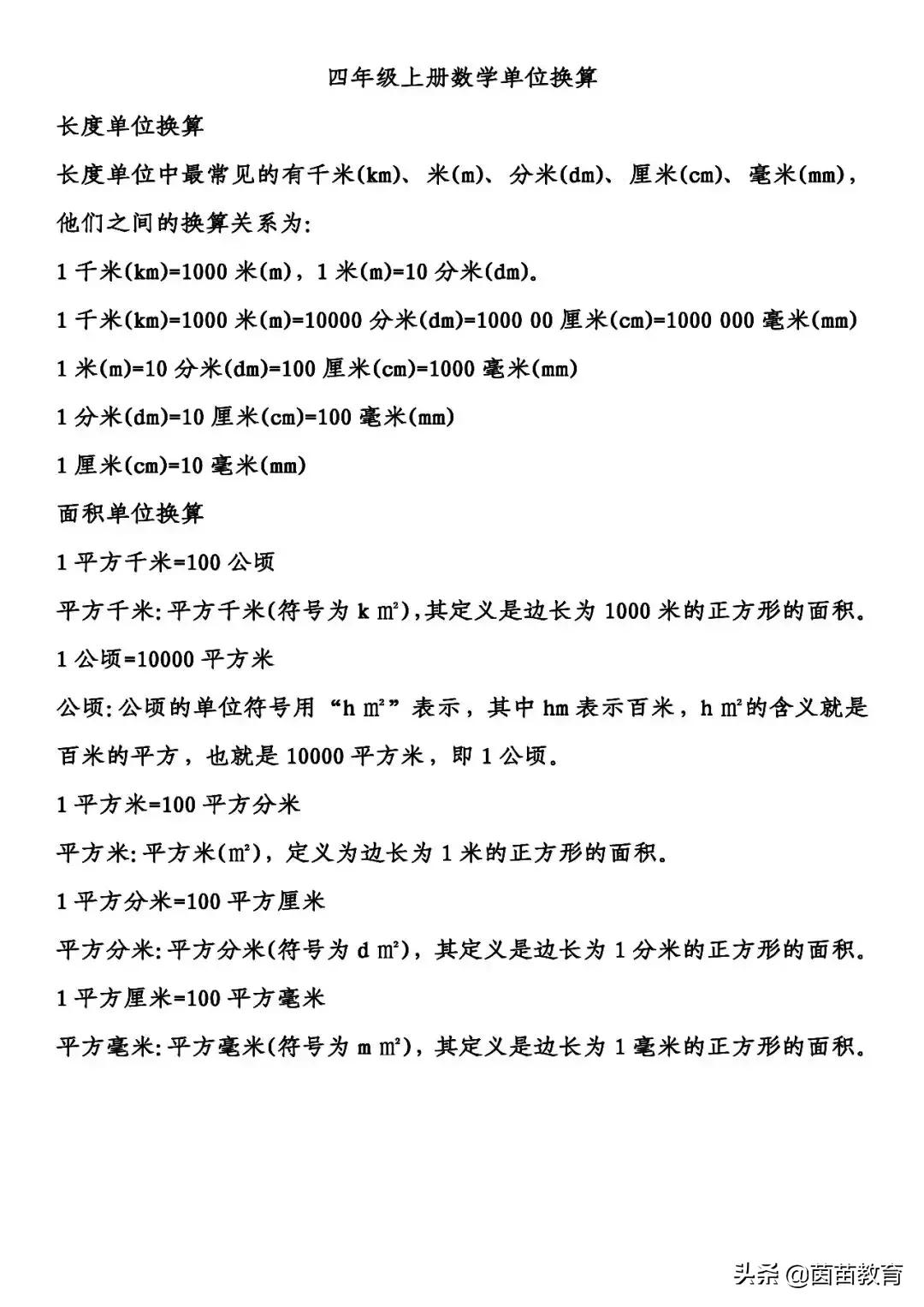# 一平方米等于多少平方厘米呢（土地面积单位怎么样才能学得扎实）

1 平方米 = 100 平方分米

1 平方分米 = 100 平方厘米

1 平方厘米 = 100 平方毫米

1 平方米 = 100 平方分米 × 100 = 10,000 平方厘米（10,000 平方厘米）

1 平方米 = 10,000 平方厘米 × 100 = 1,000,000 平方毫米（100 万平方毫米）

1 平方分米 = 100 平方厘米 × 100 = 10,000 平方毫米（10,000 平方毫米）

（一）公顷知识

100米×100米=10000平方米

1 公顷 = 1W 平方米 × 100 = 100W 平方分米

1 公顷 = 100W 平方分米 × 100 = 1 亿平方厘米

1 公顷 = 1 亿平方厘米 × 100 = 100 亿平方毫米

(2) 平方公里知识

1000米×1000米=100万平方米（100W平方米）

1平方公里=100W平方米

1平方公里=100W平方米÷10000（因为平方米和公顷的比率是1W）=100公顷